Function Repository Resource:

Calculate the radical hyperplane of two hyperspheres

Contributed by: Jan Mangaldan

## Details

The radical hyperplane is the locus of all points with the same tangential distance from the two given hyperspheres.
In 2D, the ci can be Point, Circle or Disk objects.
In 3D, the ci can be Point, Sphere or Ball objects.

## Examples

### Basic Examples (2)

Compute the radical line of two circles:

 In:=Out=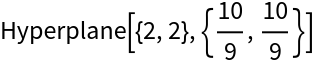Show the radical line and the two circles:

 In:=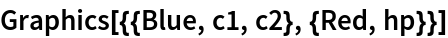Out=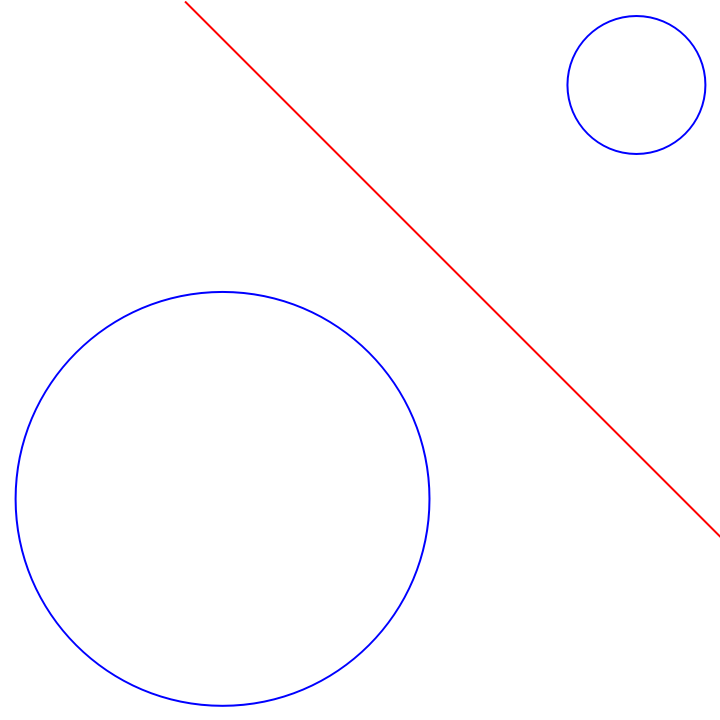Visualize the radical plane of two spheres:

 In:=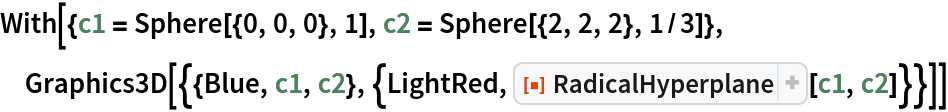Out=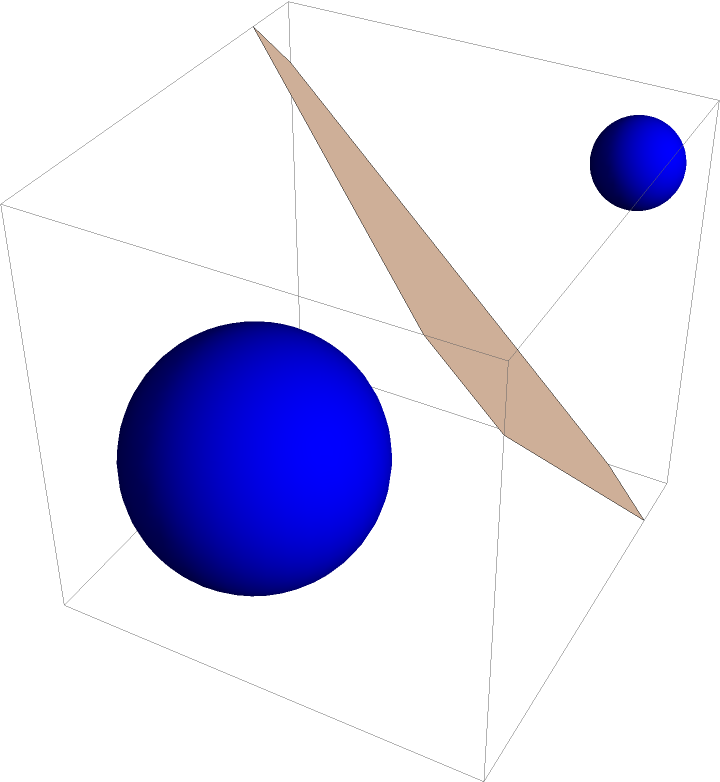### Scope (3)

The radical line of a circle and a disk:

 In:=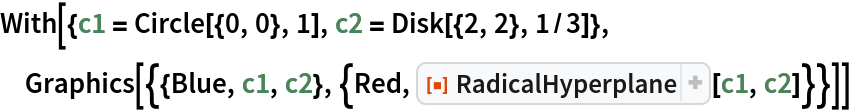Out=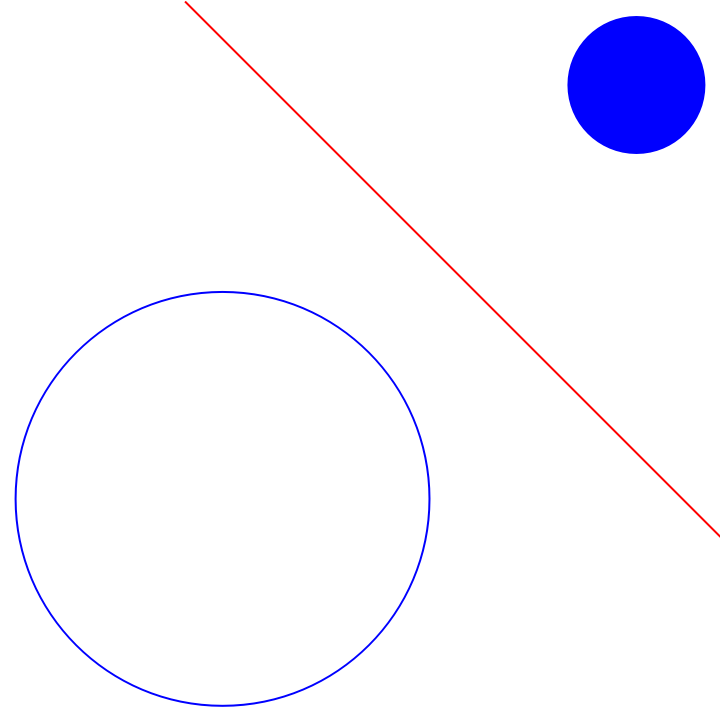The radical plane of a sphere and a ball:

 In:=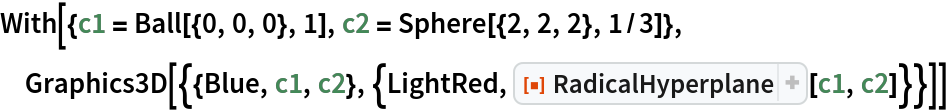Out=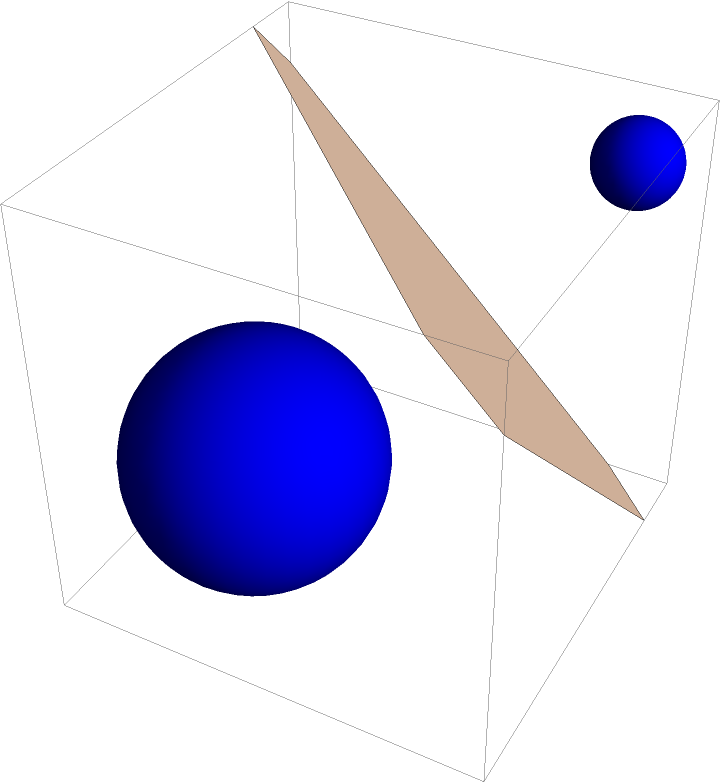A point is treated as a hypersphere with zero radius:

 In:=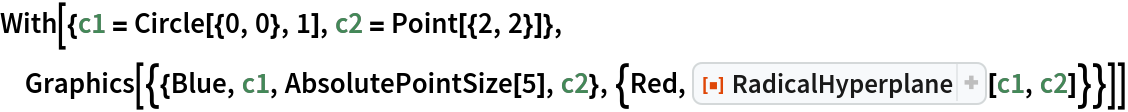Out=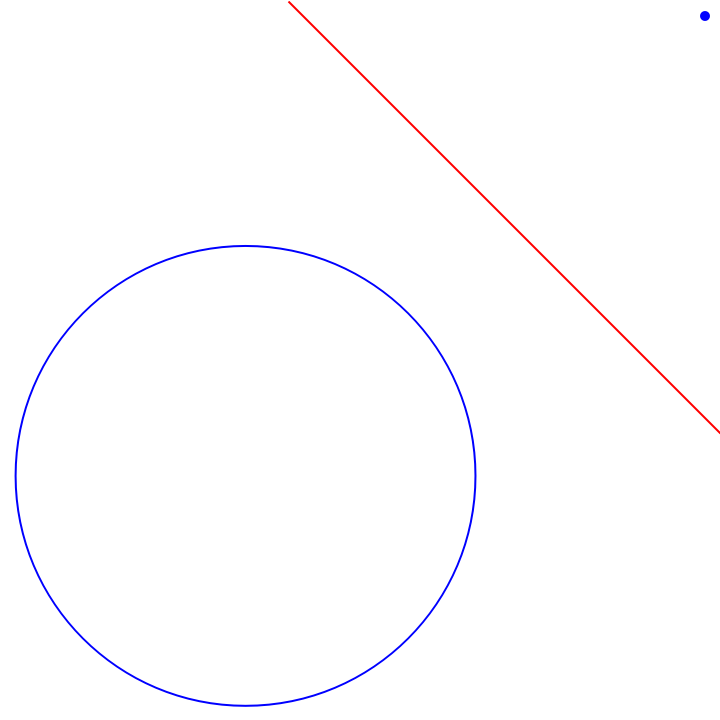### Applications (2)

Find the tangent through a given point of a circle:

 In:=Out=The radical lines of three circles with noncollinear centers and no two of them concentric, taken two at a time, coincide at the radical center:

 In:=Out=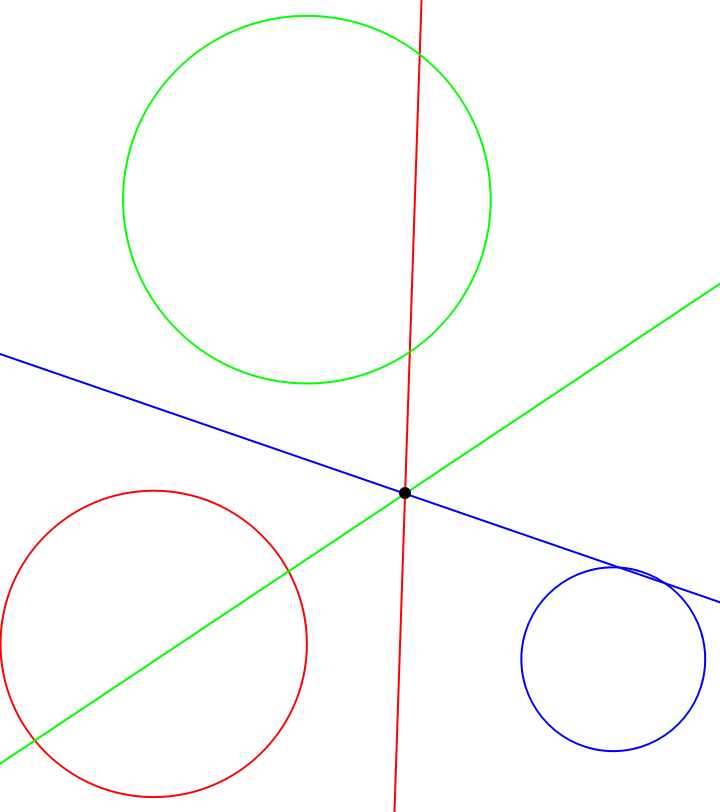### Properties and Relations (2)

If two hyperspheres intersect, the radical hyperplane passes through their intersection:

 In:=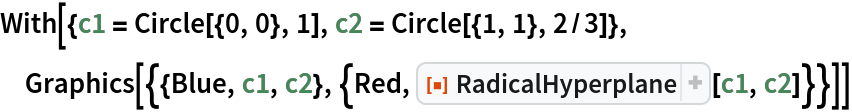Out=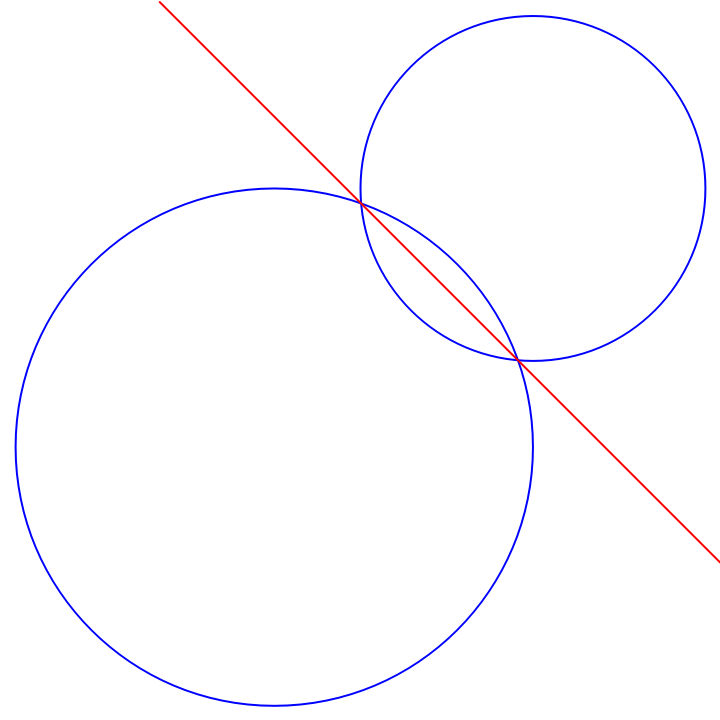Again in three dimensions:

 In:=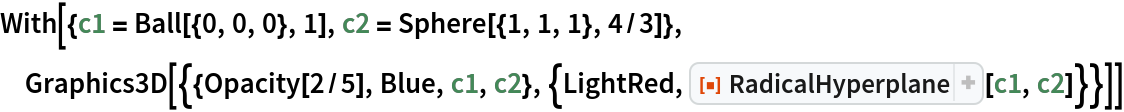Out=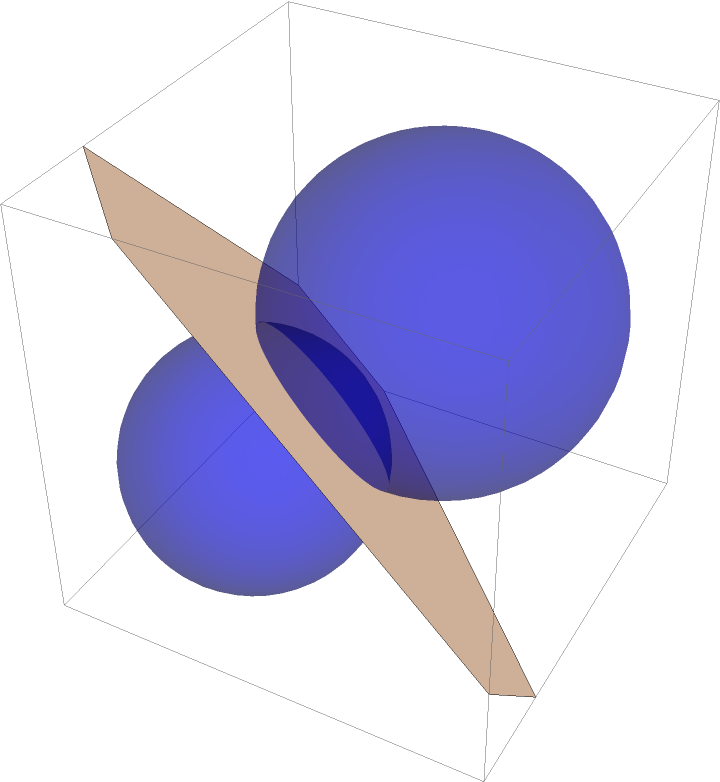The power diagram of two circles is comprised of two half-planes separated by the radical line of the two circles:

 In:=Out=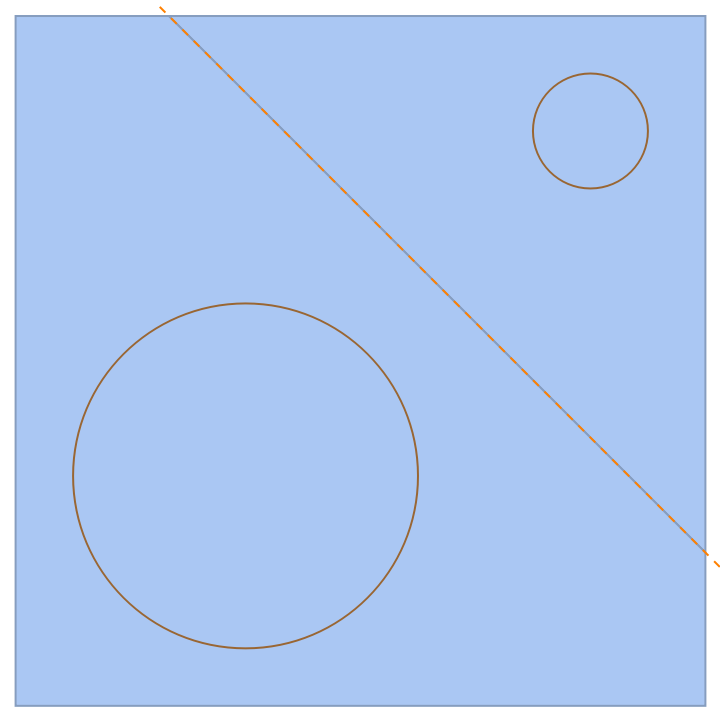### Possible Issues (1)

RadicalHyperplane is left unevaluated for concentric hyperspheres:

 In:=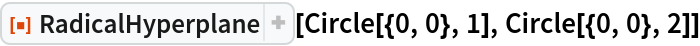Out=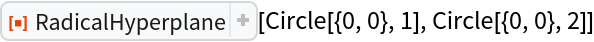### Neat Examples (1)

The pairwise radical hyperplanes of four three-dimensional, nonconcentric spheres, with all centers noncoplanar, intersect at a point:

 In:=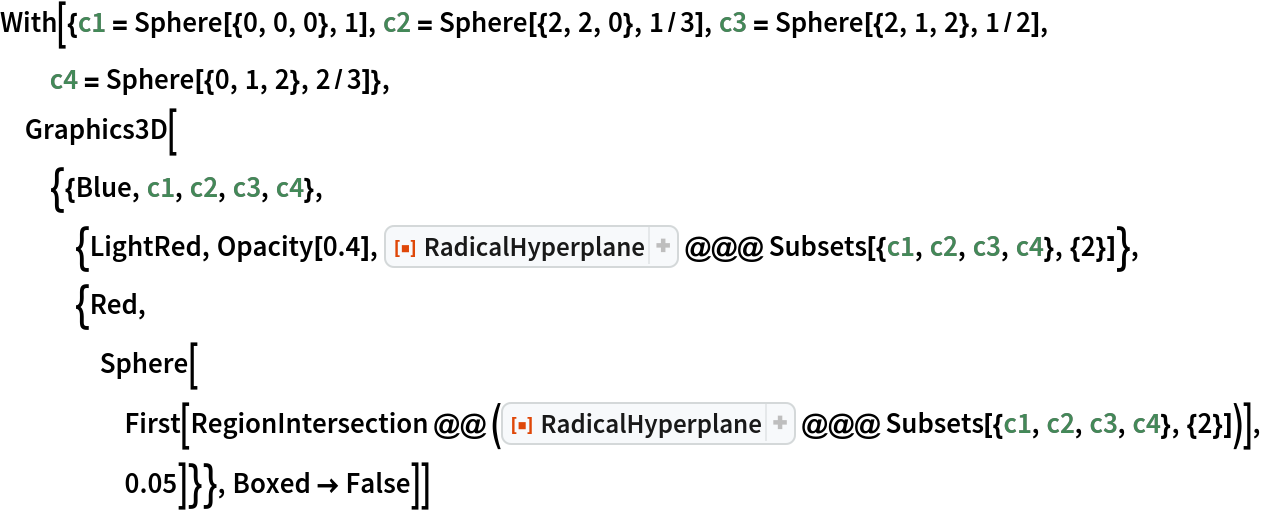Out=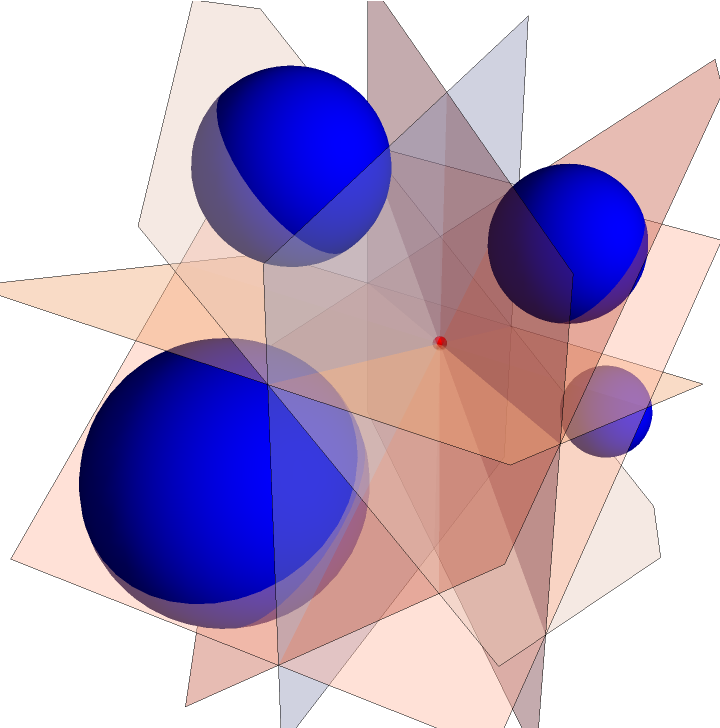## Version History

• 1.0.1 – 27 October 2021